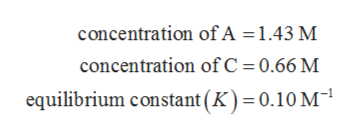# For the reaction: A + B <=> CThe concentraton of A is 1.43 M, the concentration of C is 0.66M, and the equilibrium constant is 0.10M-1.What is the concentration of B?

Question

For the reaction: A + B <=> C

The concentraton of A is 1.43 M, the concentration of C is 0.66M, and the equilibrium constant is 0.10M-1.

What is the concentration of B?

check_circleExpert Solution
Step 1

The values are given as follows:help_outlineImage Transcriptioncloseconcentration of A = 1.43 M concentration of C 0.66 M equilibrium constant (K) = 0.10 M fullscreen
Step 2

The reaction is given by as follows:

Step 3

The equilibrium constant (K) for the given ...

### Want to see the full answer?

See Solution

#### Want to see this answer and more?

Solutions are written by subject experts who are available 24/7. Questions are typically answered within 1 hour*

See Solution
*Response times may vary by subject and question
Tagged in

### Equilibrium Concepts